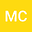•We can describe the norm for an operator given as $T:X\rightarrow Y$ as follows: It is the most appropriate value of $U$ that satisfies the following inequality $$\Vert Tx\Vert_{Y}\leq U\Vert x\Vert_{X}$$ and also for the lower bound of $T$ we can say that the value of $L$ agrees with the following inequality $$\Vert Tx\Vert_{Y}\geq L\Vert x\Vert_{X},$$ where $\Vert .\Vert_{X}$ and $\Vert .\Vert_{Y}$ stand for the norms corresponding to the spaces $X$ and $Y$. The main feature of this article is that it converts the norms and lower bounds of those matrix operators used as weighted sequence space $\ell_p(w)$ into a new space. This new sequence space is the generalized weighted sequence space. For this purpose, the double sequential band matrix $\tilde{B}(\tilde{r},\tilde{s})$ and also the space consisting of those sequences whose $\tilde{B}(\tilde{r},\tilde{s})$ transforms lie inside $\ell_p(\tilde{w})$, where $\tilde{r}=(r_{n})$, $\tilde{s}=(s_{n})$ are convergent sequences of positive real numbers. When comparing with the corresponding results in the literature, it can be seen that the results of the present study are more general and comprehensive.